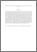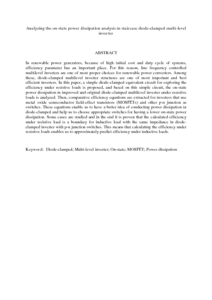# Analyzing the on-state power dissipation analysis in staircase diode-clamped multi-level inverter

## Citation

Esfandiari, Ehsan and Mariun, Norman and Marhaban, Mohammad Hamiruce and Zakaria, Azmi (2010) Analyzing the on-state power dissipation analysis in staircase diode-clamped multi-level inverter. IEICE Transactions on Electronics, E93.C (12). pp. 1670-1678. ISSN 0916-8524; ESSN: 1745-1353

## Abstract

In renewable power generators, because of high initial cost and duty cycle of systems, efficiency parameter has an important place. For this reason, line frequency controlled multilevel inverters are one of most proper choices for renewable power converters. Among these, diode-clamped multilevel inverter structures are one of most important and best efficient inverters. In this paper, a simple diode-clamped equivalent circuit for exploring the efficiency under resistive loads is proposed, and based on this simple circuit, the on-state power dissipation in improved and original diode-clamped multilevel inverter under resistive loads is analyzed. Then, comparative efficiency equations are extracted for inverters that use metal oxide semiconductor field-effect transistors (MOSFETs) and other p-n junction as switches. These equations enable us to have a better idea of conducting power dissipation in diode-clamped and help us to choose appropriate switches for having a lower on-state power dissipation. Some cases are studied and in the end it is proven that the calculated efficiency under resistive load is a boundary for inductive load with the same impedance in diode-clamped inverter with p-n junction switches. This means that calculating the efficiency under resistive loads enables us to approximately predict efficiency under inductive loads.Preview
PDF (Abstract)
Analyzing the on.pdfView Item